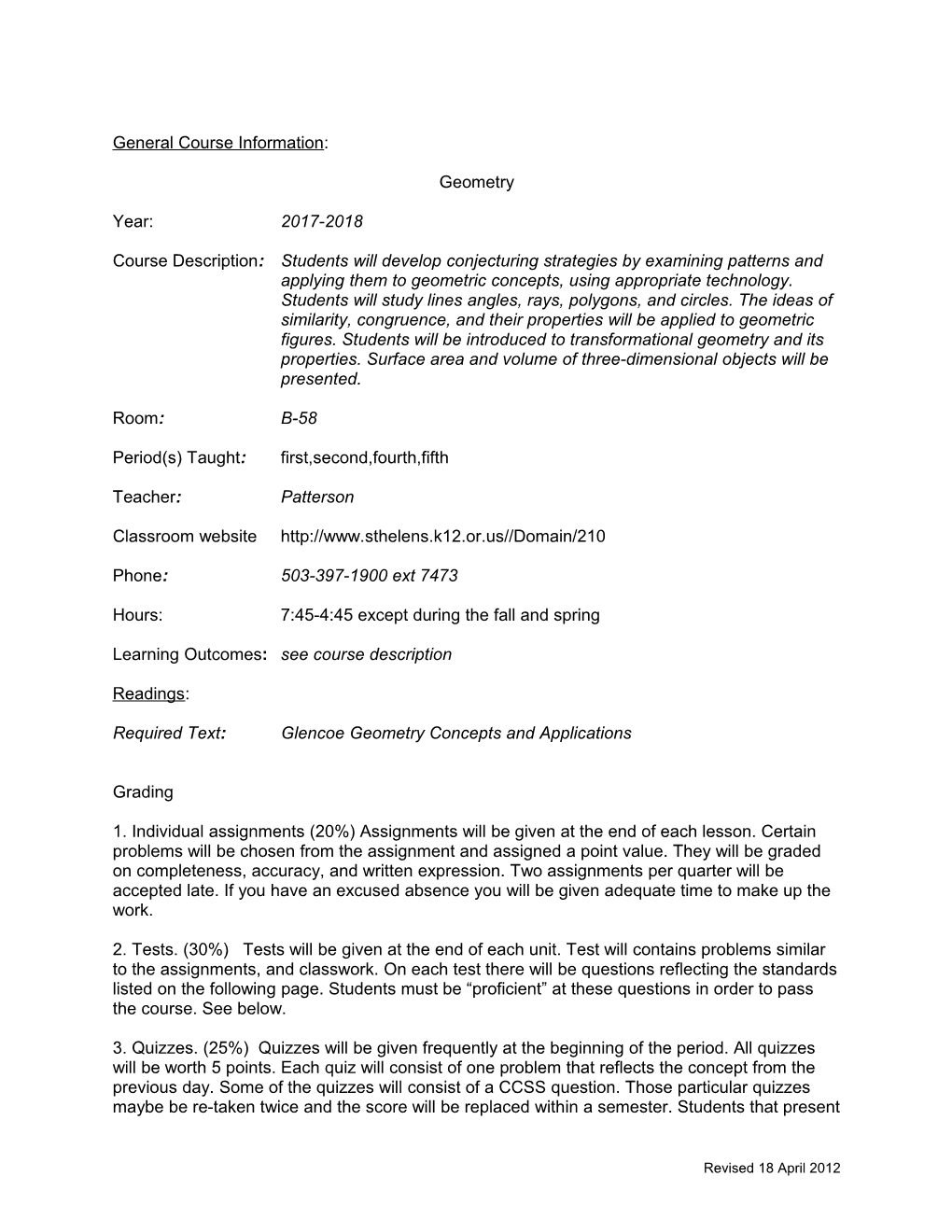# Period(S) Taught:First,Second,Fourth,FifthGeneral Course Information:

Geometry

Year:2017-2018

Course Description:Students will develop conjecturing strategies by examining patterns and applying them to geometric concepts, using appropriate technology. Students will study lines angles, rays, polygons, and circles. The ideas of similarity, congruence, and their properties will be applied to geometric figures. Students will be introduced to transformational geometry and its properties. Surface area and volume of three-dimensional objects will be presented.

Room:B-58

Period(s) Taught:first,second,fourth,fifth

Teacher: Patterson

Classroom website

Phone:503-397-1900 ext7473

Hours:7:45-4:45 except during the fall and spring

Learning Outcomes:see course description

Required Text:Glencoe Geometry Concepts and Applications

1. Individual assignments (20%) Assignments will be given at the end of each lesson. Certain problems will be chosen from the assignment and assigned a point value. They will be graded on completeness, accuracy, and written expression. Two assignments per quarter will be accepted late. If you have an excused absence you will be given adequate time to make up the work.

2. Tests. (30%) Tests will be given at the end of each unit. Test will contains problems similar to the assignments, and classwork. On each test there will be questions reflecting the standards listed on the following page. Students must be “proficient” at these questions in order to pass the course. See below.

3. Quizzes. (25%) Quizzes will be given frequently at the beginning of the period. All quizzes will be worth 5 points. Each quiz will consist of one problem that reflects the concept from the previous day. Some of the quizzes will consist of a CCSS question. Those particular quizzes maybe be re-taken twice and the score will be replaced within a semester. Students that present their response to the quiz will earn no less than a 4, if they have made a legitimate effort towards answering the quiz.

4. Projects. (20%) There will be projects assigned for some of the units. You will be given a few weeks to complete them. Some class time maybe given. Projects can be done in groups of less than three, but each person is responsible for their own write up and follow up. If the write-ups appear to be similar, both project write-ups will be returned to the students the next class day. The students will then have one day to return the write-ups to me and two points will be deducted from the score. Projects turned in late will receive 80% credit.

5. Educational Task Grade – 2pts. (5%) Each day you will be given two points, if you perform the task of the day i.e. do the explorations or tasks, then you will keep the two points otherwise you will lose them.

6. Notebooks 3 percentage points per quarter. I recommend you take notes on ideas presented to you. I will give you directions on what I think is needed in your notebook. At the end of each quarter if your notebook is complete with examples, ideas, and concepts we discuss in class, you will earn three percentage points. Certain lessons will require the cornell note taking style, to earn the full 3 points you must have completed the cornell style notes

7. A semester and a final exam will be used as a tie breaker to determine semester grade for the course, if it does not serve as a tie breaker then it will be worth 15% of the semester grade.

Common Core State Standards (CCSS)

These are the skills you will need to demonstrate understanding on in order to pass the course. They will appear in tests and quizzes and will be noted as a CCSS standard. Students must earn a score of “proficiency” in 8 of 12 essential skills. Students will be given three opportunities as necessary to demonstrate proficiency in these CCSS in the during the semester in which the CCSS is assessed.

Redoing CCSS:

A student may request to redo a quiz that contains the CCSS within the semester. The passing score will replace the original quiz score as long as the CCSS quiz is done within the semester it was assigned.

What is “Proficiency”

As defined in the school district grading polices and procedures handbook proficiency is the student demonstrates consistent understanding of the course standards.

Geometry CCSS Essential Skills

Semester 1

1. Define the following vocabulary: Angle, Circle, Perpendicular Lines, Parallel lines, Line segment
2. Know parallel lines have equal slope and perpendicular lines have negative reciprocal slope.
3. Given a polygon, sketch a translation, reflections, rotation, and dilation
4. Determine whether two triangles are congruent using the 4 congruent triangle postulates.
5. Use Pythagorean Theorem to solve applied problems
6. Identify, describe, and solve problems using properties of Quadrilaterals

Semester 2

1. Identify and solve problems using similarity (missing measures, scale factors, etc.)
2. Use trigonometric ratios to solve applied problems
3. Identify and solve surface area and volume of 3-dimensional solids
4. Know angles relationships in circles (central angles, inscribed angles, etc.)
5. Know relationships of line segments in circles (radii, chords, tangents, etc.)
6. Perform translations, reflections, rotations, and dilations on a coordinate plane

Revised 18 April 2012